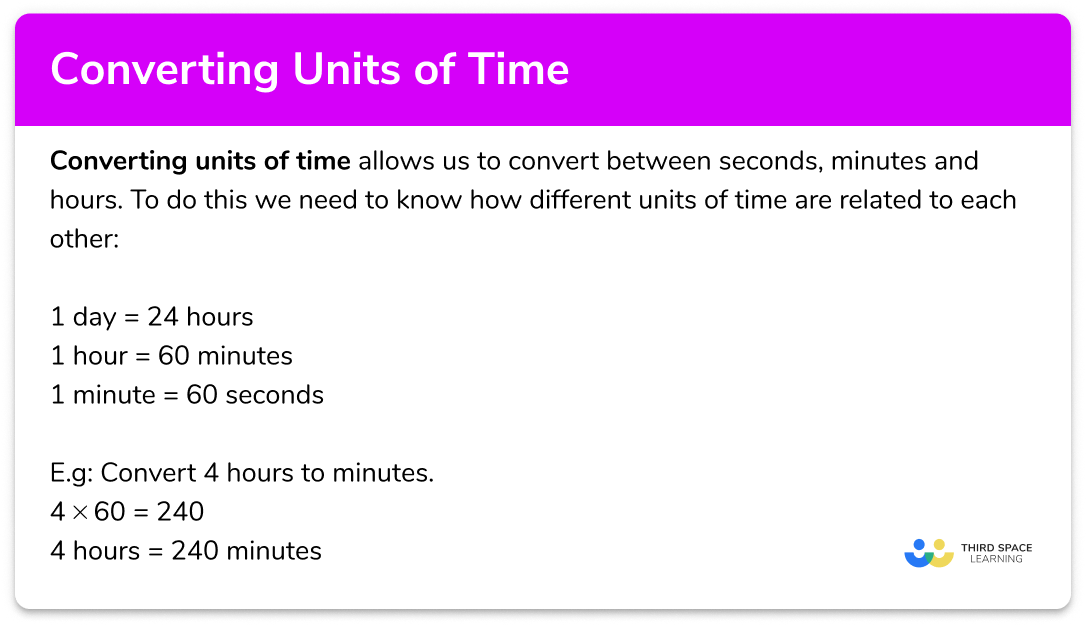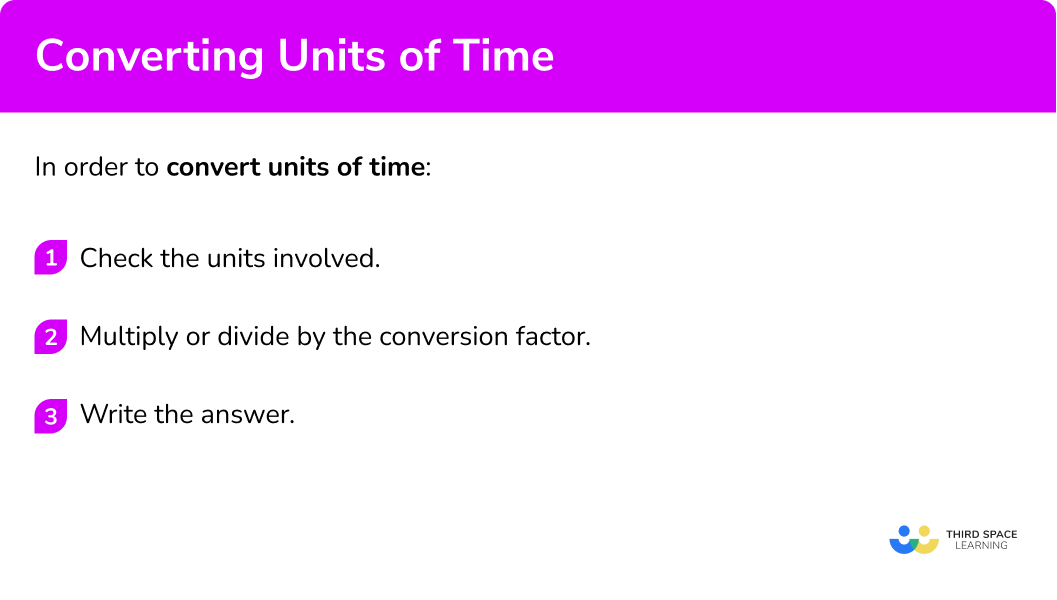# Converting Units Of Time

Here we will learn about converting units of time, including converting between seconds, minutes and hours.

There are also worksheets based on Edexcel, AQA and OCR exam questions, along with further guidance on where to go next if you’re still stuck.

## What is converting units of time?

Converting units of time is converting between seconds, minutes and hours.

To do this we need to know how different units of time are related to each other:

1 day = 24 hours

1 hour = 60 minutes

1 minute = 60 seconds

E.g

Convert 4 hours to minutes.

4 \times 60=240

4 hours = 240 minutes

### What is converting units of time?## How to convert units of time

In order to convert units of time:

1. Check the units involved.
2. Multiply or divide by the conversion factor.

### How to convert units of time## Related lessons on units of measurement

Converting units of time is part of our series of lessons to support revision on units of measurement. You may find it helpful to start with the main units of measurement lesson for a summary of what to expect, or use the step by step guides below for further detail on individual topics. Other lessons in this series include:

## Converting units of time examples

### Example 1: days to hours

How many hours are there in 1.5 days?

1. Check the units involved.

1 day = 24 hours

So the conversion factor is 24.

2Multiply or divide by the conversion factor.

We need to multiply by 24.

1.5 \times 24=36

1.5 days is 36 hours

### Example 2: hours to minutes

How many minutes are there in 2.3 hours?

Check the units involved.

Multiply or divide by the conversion factor.

### Example 3: hours >>> hours and minutes

Convert 1.4 hours to hours and minutes.

Check the units involved.

Multiply or divide by the conversion factor.

### Example 4: minutes to hours

Convert 324 minutes to hours.

Check the units involved.

Multiply or divide by the conversion factor.

### Example 5: minutes >>> hours and minutes

Convert 520 minutes to hours and minutes

Check the units involved.

Multiply or divide by the conversion factor.

### Example 6: hours and minutes  >>> hours

Convert 2 hours and 45 minutes to hours.

Check the units involved.

Multiply or divide by the conversion factor.

### Common misconceptions

• Base \bf{10} and base \bf{60}

A common misconception is to confuse the units used in the metric system with the units used for time.

The metric system uses base 10 .

Each successive unit is 10 times larger than the previous one.

E.g.

Time uses base 60.

E.g.

### Practice converting units of time questions

1. How many hours are there in 2\frac{1}{2} days?

60 hours48 hours72 hours63 hours2\frac{1}{2}\times 24=60

2. How many minutes are there in 3.1 hours?

31 minutes190 minutes186 minutes310 minutes3.1\times 60=186

3. Convert 4.35 hours to hours and minutes?

4 hours and 35 minutes4 hours and 25 minutes4 hours and 21 minutes4 hours and 28 minutesConcentrate on the decimal fraction part

0.35\times 60=21

So, 4.35 hours is 4 hours and 21 minutes.

4. Convert 75 minutes to hours?

0.75 hours1.5 hours minutes7.5 hours1.25 hours75\div 60=1.25

5. Convert 135 minutes to hours and minutes?

13 hours and 5 minutes1 hours and 35 minutes2 hours and 25 minutes2 hours and 15 minutes135\div 60=2.25

So there are 2 hours.

0.25\times 60=15

And there are 15 minutes

6. Convert 4 hours and 18 minutes to hours?

4.3 hours4.18 hours4.2 hours4.81 hoursFirst look at the minutes

18 \div 60=0.3

So the minutes are 0.3 hours.

Altogether there are 4.3 hours.

### Converting units of time GCSE questions

1. How many days are 120 hours?

A             B            C           D

(1 mark)

120\div 24=5

C – 5 hours

(1)

2. (a) Write 102 minutes as hours

(b) Write 2 hours and 48 minutes as hours.

(4 marks)

(a)

102\div 60

(1)

1.7 hours

(1)

(b)

48\div 60=0.8

(1)

2.8 hours

(1)

3. Work out how many seconds there are in 3 hours.

(2 marks)

3\times 60 \times 60

(1)

10 \ 800 seconds

(1)

## Learning checklist

You have now learned how to:

• Change between standard units of time

## Still stuck?

Prepare your KS4 students for maths GCSEs success with Third Space Learning. Weekly online one to one GCSE maths revision lessons delivered by expert maths tutors.

Find out more about our GCSE maths tuition programme.